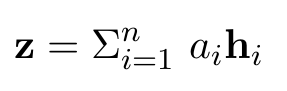# Regularisation of dense output layer does not seem to work as expected

I have a neural network that I pretrain on Dataset A and then finetune on Dataset B - before finetuning I add a dense layer on top of the model (red arrow) that I would like to regularise. However, it does not seem to work properly: either the performance drops very low even with tiny regularisation weights (0.01 - 0.08 weight range, f1 drops from around 22% to 12% on the dev set) or I get the exact same F1 score for all weights (as if I train the same model several times with no changes). <-- this does not occur anymore

(Since new users can only post one image for whatever reason, I have to resort to a screenshot here…)

Reg. layer initialisation:
`self.reg_matrix =` `torch.cat((torch.eye(42), torch.zeros(42, 200)), dim=1).cuda()`

Reg. term (adapt is the dense layer W):
`squared_sum = torch.sub(self.model.adapt.weight, self.reg_matrix).pow(2).sum()`

Loss:
`loss += self.weight * squared_sum`

I originally assumed that the tape wasn’t recording all operations properly since I hadn’t used PyTorch operations for the calculation of the L2 reg. term, but even when I do it doesn’t seem to work.

This is the function I’m adapting, so the previous code is around line 42 here (loss function is unchanged):

Results (F1 (micro)):

``````0.0 => 22.361%
0.01 => 13.309%
0.05 => 11.350%
1.0 => 12.084%
``````

F1 scores from evaluation would be something like 42% with 0.0 and around 17% to 13% or so with regularisation. The results of the original model without pretraining is 65%.

Any ideas would be appreciated.

Could you explain how the regularization term is working?
Currently it seems you are subtracting a matrix of the shape `[42, 242]` with 42 ones in the first diagonal from the weight before squaring and summing it.
Usually you would add the weight norm to the loss and I’m unfamiliar with your approach.
Did you try to use the standard weight decay?

That is exactly the idea, yes. This regularisation is supposed to keep the weights of the new dense layer close to the identity matrix (in the regularisation matrix) so that with increasing reg. weight the output of the previous dense layer is not transformed much. I realise now that I could have explained the core idea better.
For this you can just assume that the regularisation is correct. How do I regularise with a custom regularisation scheme like this?

That doesn’t seem to offer me a way to incorporate the regularisation matrix if I understand it correctly.

More detail:

1. I pretrain the model with data A: [emb -> LSTM -> attention -> dense -> softmax]
2. Afterwards I freeze all the ‘old’ layers and add a dense layer after the original dense (output) layer, so now it is [emb -> LSTM -> attention -> dense -> dense -> softmax], the new dense layer has the dimensions of the original output dense layer and the LSTM layer combined: so dense1(42, 42) + lstm(42, 200) = dense2(42, 242)
3. Now, while finetuning the new dense layer receives the output of the original output dense layer and the output of the lstm, so: dense2([dense1();lstm()]) -> softmax
4. The weights of this new dense layer are now supposed to be regularised through the formula that I posted (controlled by the reg. weight)
5. For this to work I need to add this term to the loss (or at least that is what I have done so far, is there another way in PyTorch?):I’m still unsure if your approach is right.
Note that you are subtracting a constant tensor with 1s at some positions from `self.model.adapt.weight`, while it seems your approach would rather subtract the values of `self.weight` at these positions from `self.model.adapt.weight`?

If you are convinced that the formula is right and I’m misunderstanding the use case, then the approach should work. You could just add the regularization loss to the final loss and call `backward` on it.

1 Like

It is, I just explained it badly.That’s what I’m doing; at least now I know that I’m not misunderstanding PyTorch itself in this case.

Thank you!

1 Like